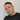# Chi-square test in SPSS

May 12, 2019

In this quick tutorial we’ll look at performing a chi-square test on a single sample. More specifically, we’ll look into performing a hypothesis test on a categorical variable in order to determine if the distribution of categories is about the same. So the null hypothesis would assume that the probabilties of all categories do not significantly differ from each other.

For this purpose, we’ll use the demo.sav dataset and test the Income category variable which consists of 4 different categories. The null hypothesis will state that the representation of these categories is roughly equal. Go to Analyze >> Nonparametric Tests and select Automatically compare observed data to hypothesized. The confidence level defaults to 95% which is just fine in this case. In the fields tab select the Income category variable and hit Run.

We are presented with an output window which clearly states the null hypothesis assumed and the result of the test. In this case we can clearly see that the significance is very low (0.000) and consequently SPSS concludes the decisision should be to reject the null hypothesis of equal probabilites across categories.Written by Johan Osterberg who lives and works in Gothenburg, Sweden as a developer specialized in e-commerce. Connect with me on Linkedin